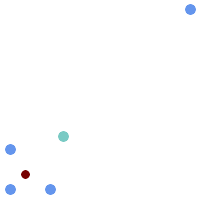## Name

ST_GeometricMedian — 返回多点的几何中位数。

## Synopsis

`geometry ST_GeometricMedian (` geometry geom, float8 tolerance = NULL, int max_iter = 10000, boolean fail_if_not_converged = false`)`;

## 描述该函数支持 3d 并且不会丢失 z-index。该功能支持M坐标。

## 示例```WITH test AS (
SELECT 'MULTIPOINT((10 10), (10 40), (40 10), (190 190))'::geometry geom)
SELECT
ST_AsText(ST_Centroid(geom)) centroid,
ST_AsText(ST_GeometricMedian(geom)) median
FROM test;

centroid      |                 median
--------------------+----------------------------------------
POINT(62.5 62.5) | POINT(25.01778421249728 25.01778421249728)
(1 row)
```